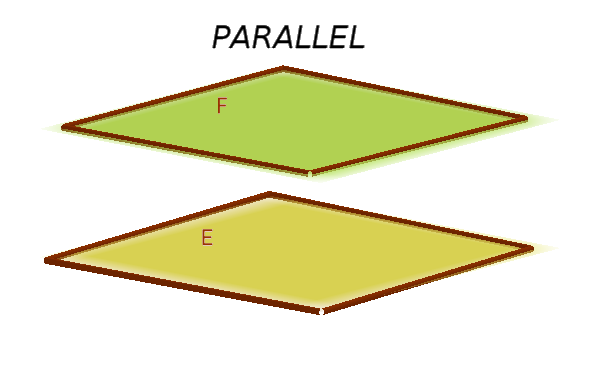Math Relative position of planes Plane and plane

# Relative position of plane and plane

A distinction is made between three possible relative positions between two planes $E$ and $F$.

• They intersect.• They are parallel.• They are coincident.!

### Remember

If two planes intersect, there is no point of intersection but a line intersection.

Similar to the relative position of a line and a plane, one tries to calculate the intersection line.
However, if you come across a true statement (e.g. 0 = 0), the planes are coincident. If the statement is incorrect (e.g. 8 = 0), they are parallel.

i

### Hint

The easiest way to calculate the intersection line is when both planes are in the cartesian form.

### Example

$\text{E: } x-y+z=2$

$\text{F: } 2x+y+z=4$

1. #### Set up a system of equations

The two equations can be viewed as a system of equations.
1. $x-y+z=2$
2. $2x+y+z=4$

Now you should eliminate a variable. This is achieved here, for example, by adding the two equations.

I.+II.

$3x+2z=6$

2. #### Replace variable with $r$

One of the other variables is now replaced by $r$ and inserted in the equation. For example x:

$\color{red}{x=r}$

$3r+2z=6$

The other variable ($z$) can now be expressed as a function of $r$. Simply solve equation for $z$.

$3r+2z=6\quad|-3r$
$2z=6-3r\quad|:2$
$\color{red}{z=3-1.5r}$

One of the two plane equations can also be used to determine $y$ by using $x$ and $z$.

$x-y+z=2$
$r-y+(3-1.5r)=2$
$-0.5r-y+3=2\quad|+y$
$-0.5r+3=2+y\quad|-2$
$\color{red}{y=-0.5r+1}$

3. #### Set up equation of a line

First we write the results for $x$, $y$ and $z$ among themselves.
1. $x=r$
2. $y=-0.5r+1$
3. $z=3-1.5r$

Sorted:

1. $x=\color{blue}{0}\color{green}{+1}r$
2. $y=\color{blue}{1}\color{green}{-0.5}r$
3. $z=\color{blue}{3}\color{green}{-1.5}r$

This can now be easily put into the form of a equation of a line.

$\vec{x} = \begin{pmatrix} \, \\ \, \\ \, \end{pmatrix} + r \cdot \begin{pmatrix} \, \\ \, \\ \, \end{pmatrix}$

$\vec{x} = \begin{pmatrix} \color{blue}{0} \\ \color{blue}{1} \\ \color{blue}{3} \end{pmatrix} + r \cdot \begin{pmatrix} \color{green}{1} \\ \color{green}{-0.5} \\ \color{green}{-1.5} \end{pmatrix}$

### Example (parallel)

$\text{E: } x-y+z=2$

$\text{F: } 2x-2y+2z=7$

1. #### Set up a system of equations

1. $x-y+z=2\,\,\,|\cdot(-2)$
2. $2x-2y+2z=7$

We apply the addition method.

1. $-2x+2y-2z=-4$
2. $2x-2y+2z=7$

I.+II.
$0=3$ f. s.

2. #### Interpret the result

We obtain a contradiction or a false statement.

$0\neq3$

$E$ and $F$ therefore have no common point. The planes must be parallel.

=> $E$ and $F$ are parallel

i

### Hint

Two parallel planes can also be recognized by the fact that the normal vectors of the planes are multiples of one another (collinear).